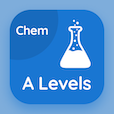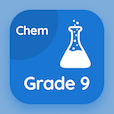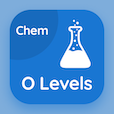Online A Level Courses

A Level Chemistry Practice Tests

A Level Chemistry Tests

The Book Mole Calculations Multiple Choice Questions (MCQ Quiz) PDF, Mole Calculations MCQs download to study online a level chemistry certification courses. Study Moles and Equations Multiple Choice Questions and Answers (MCQs), Mole Calculations quiz answers PDF for schools that offer certificate programs. The eBook Mole Calculations MCQ App Download: atoms and molecules mass, solutions and concentrations, gas volumes, mole calculations test prep for colleges that offer online courses.

The MCQ: The ratio of hydrogen to oxygen in a water molecule is equal to PDF, Mole Calculations App Download (Free) with 1:2 ratio, 2:1 ratio, 4:3 ratio, and 1:4 ratio choices for schools that offer certificate programs. Practice mole calculations quiz questions, download Amazon eBook (Free Sample) for schools that offer online bachelor degrees.

## Chemistry: Mole Calculations MCQs

MCQ: The ratio of hydrogen to oxygen in a water molecule is equal to

A) 1:2 ratio
B) 2:1 ratio
C) 4:3 ratio
D) 1:4 ratio

MCQ: The molecular formula of different compounds shows actual numbers of

A) atoms
B) molecules
C) moles
D) ions

MCQ: Two Greek words 'element' and 'measure' constitutes the word

A) spectrometry
B) stoichiometry
C) elemetry
D) geometry

MCQ: The percentage by mass of any element is calculated with the help of

A) formula of compound only
B) relative atomic masses only
C) nucleon number
D) formula of compound and relative atomic masses

MCQ: The mass of each element in a compound helps in determining

A) empirical formula
B) molecular formula
C) structural formula
D) model

### Practice Tests: A Level Chemistry Exam Prep

Download A level Chemistry Quiz App, 9th Grade Chemistry MCQ App, and O Level Chemistry MCQs App to install for Android & iOS devices. These Apps include complete analytics of real time attempts with interactive assessments. Download Play Store & App Store Apps & Enjoy 100% functionality with subscriptions!